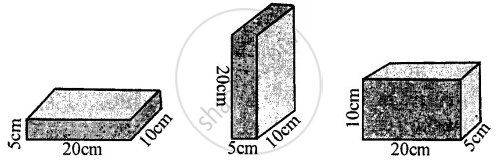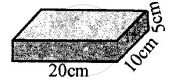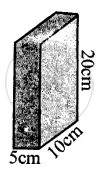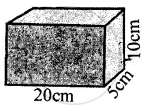ICSE Class 8CISCE
Share

# Figure Shows a Brick of Weight 2 Kgf and Dimensions 20 Cm X 10 Cm × 5 Cm Placed in Three Different Positions on the Ground. Find the Pressure Exerted by the Brick in Each Case. - ICSE Class 8 - Physics

ConceptPressure - Calculation of Pressure in Simple Cases

#### Question

Figure shows a brick of weight 2 kgf and dimensions 20 cm x 10 cm × 5 cm placed in three different positions on the ground. Find the pressure exerted by the brick in each case.#### Solution

(1) Weight of brick = Thrust = F = 2 kgfArea of base = 20/100xx10/100=1/50m^2

Pressure exerted P =F/A =(2kgf)/(1/50)m^2 = 100kgf m^-2

Area of base = area of top L × B
20 Cm × 10 = 200 cm2

P= (Thrust)/A= (2kgf)/(200cm^2)=1/100 = 0.01kgf cm^-2

(2)Area of base = area of top

= 5cm xx 10 cm = 50 cm^2

Pressure exerted = P = F/A =( 2kgf)/ (50 cm^2)= 0.04

P = 0.04 kgf cm^-2

(3) Weight of brick
F = 2 kgf
Area of base = L × B
= 20 cm × 5 cm = 100 cm2Pressure exerted =F/A = 2/100=0.02 kgf cm^-2

Is there an error in this question or solution?
Solution Figure Shows a Brick of Weight 2 Kgf and Dimensions 20 Cm X 10 Cm × 5 Cm Placed in Three Different Positions on the Ground. Find the Pressure Exerted by the Brick in Each Case. Concept: Pressure - Calculation of Pressure in Simple Cases.
S